# CBSE Physics for Class 12 | Electrostatics | Electric Lines of Force

## Electric Lines of Force

### (1) Definition :

The electric field in a region is represented by continuous lines (also called lines of force). Field line is an imaginary line along which a positive test charge will move if left free.

Electric lines of force due to an isolated positive charge, isolated negative charge and due to a pair of charge are shown below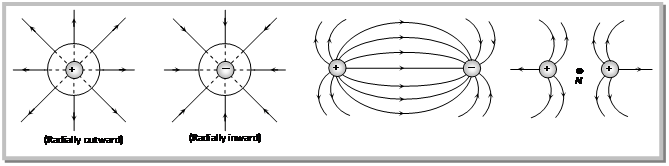### (2) Properties of electric lines of force

(i) Electric field lines come out of positive charge and go into the negative charge.

(ii) Tangent to the field line at any point gives the direction of the field at that point.(iii) Field lines never cross each other.

(iv) Field lines are always normal to conducting surface.(v) Field lines do not exist inside a conductor.

(vi) The electric field lines never form closed loops. (While magnetic lines of forces form closed loop)(vii) The number of lines originating or terminating on a charge is proportional to the magnitude of charge. In the following figure electric lines of force are originating from A and terminating at B hence QA is positive while QB is negative, also number of electric lines at force linked with QA are more than those linked with QB hence(viii) Number of lines of force per unit area normal to the area at a point represents magnitude of intensity (concept of electric flux i.e.,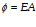)

(ix) If the lines of forces are equidistant and parallel straight lines the field is uniform and if either lines of force are not equidistant or straight line or both the field will be non uniform, also the density of field lines is proportional to the strength of the electric field. For example see the following figures.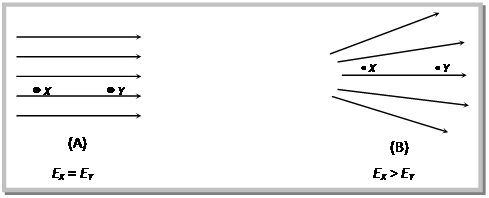### (3) Electrostatic shielding :

Electrostatic shielding/screening is the phenomenon of protecting a certain region of space from external electric field. Sensitive instruments and appliances are affected seriously with strong external electrostatic fields. Their working suffers and they may start misbehaving under the effect of unwanted fields.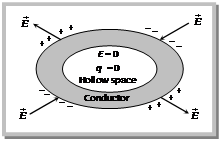The electrostatic shielding can be achieved by protecting and enclosing the sensitive instruments inside a hollow conductor because inside hollow conductors, electric fields is zero.

(i) It is for this reason that it is safer to sit in a car or a bus during lightening rather than to stand under a tree or on the open ground.

(ii) A high voltage generator is usually enclosed in such a cage which is earthen. This would prevent the electrostatic field of the generator from spreading out of the cage.

(iii) An earthed conductor also acts as a screen against the electric field. When conductor is not earthed field of the charged body C due to electrostatic induction continues beyond AB. If AB is earthed, induced positive charge neutralizes and the field in the region beyond AB disappears.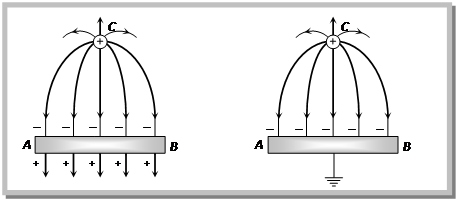# CBSE Physics for Class 12 | Electrostatics | Electric Potential

## Electric Potential

### (1) Definition of Electric Potential.:

Potential at a point in a field is defined as the amount of work done in bringing a unit positive test charge, from infinity to that point along any arbitrary path (infinity is point of zero potential). Electric potential is a scalar quantity, it is denoted by V;$\displaystyle V=\frac{W}{{{q}_{\mathbf{0}}}}$

### (2) Unit and dimensional formula of Electric Potential.:

S. I. unit –$\displaystyle \frac{Joule}{Coulomb}=volt$ C.G.S. unit – Stat volt (e.s.u.); 1 volt$\displaystyle =\frac{\mathbf{1}}{\mathbf{300}}$ Stat volt Dimension of electric potential are$\displaystyle [V]=[M{{L}^{2}}{{T}^{-3}}{{A}^{-1}}]$

### (3) Types of electric potential :

According to the nature of charge potential is of two types

(i) Positive potential : Due to positive charge.

(ii) Negative potential : Due to negative charge.

### (4) Potential of a system of point charges :

Consider P is a point at which net electric potential is to be determined due to several charges. So net potential at P$\displaystyle V=k\frac{{{Q}_{1}}}{{{r}_{1}}}+k\frac{{{Q}_{2}}}{{{r}_{2}}}+k\frac{{{Q}_{3}}}{{{r}_{3}}}+k\frac{\left( -{{Q}_{4}} \right)}{{{r}_{4}}}+...$

In general$\displaystyle V=\sum\limits_{i=1}^{X}{\frac{k{{Q}_{i}}}{{{r}_{i}}}}$

Note :

• At the centre of two equal and opposite charge V = 0
but$\displaystyle E\ne 0$
• At the centre of the line joining two equal and similar charge$\displaystyle V\ne 0,\,E=0$

### (5) Electric potential due to a continuous charge distribution :

The potential due to a continuous charge distribution is the sum of potentials of all the infinitesimal charge elements in which the distribution may be divided i.e.,$\displaystyle V=\int{dV},\,\,\,\,=\int{\frac{dQ}{4\pi {{\varepsilon }_{0}}r}}$

### (6) Graphical representation of electric potential :

When we move from a positive charge towards an equal negative charge along the line joining the two then initially potential decreases in magnitude and at centre become zero, but this potential is throughout positive because when we are nearer to positive charge, overall potential must be positive.

When we move from centre towards the negative charge then though potential remain always negative but increases in magnitude fig. (A).

As one move from one charge to other when both charges are like, the potential first decreases, at centre become minimum and then increases Fig. (B).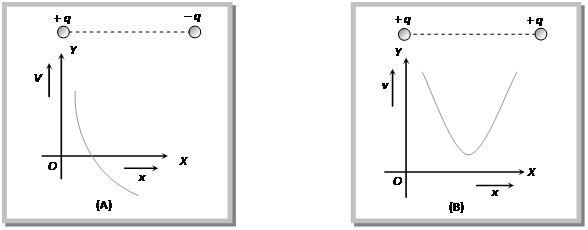### (7) Potential difference :

In an electric field potential difference between two points A and B is defined as equal to the amount of work done (by external agent) in moving a unit positive charge from point A to point B.

i.e.,$\displaystyle {{V}_{B}}-{{V}_{A}}=\frac{W}{{{q}_{0}}}$ in general$\displaystyle W=Q.\,\Delta V$;$\displaystyle \Delta V=$Potential difference through which charge Q moves.